Support Union Test Prep

Support Now

# Page 1-Geometry Study Guide for the Math Basics

## How to Prepare for the Geometry Questions on a Math Test

### General Information

Geometry is just a fancy name for the study of lines and shapes. There are certainly additional difficult principles and topics, but we have the basics here. You’ll need to master these before tackling more advanced concepts.

(Please note that if a figure is labeled “example” in this guide, it may not be the only representation of that figure, but is one of them.)

### Points and Lines

A point in geometry is a location. It has no length, width, or depth. We use a dot and a capital letter to show a point.

A line segment is defined by two points and all the points between them. The two points are called endpoints of the segment. A segment has one dimension: length. To write a segment, place a bar over the two endpoints. $$\overline{AB}$$ or $$\overline{BA}$$ is this segment:A line extends in both directions without end. To write a line, place a bar with two arrows over any two points of the line. $$\overleftrightarrow{AB}$$ or $$\overleftrightarrow{BA}$$ is the line: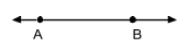A ray starts at one endpoint and extends without end in only one direction. To write a ray, place a bar with an arrow pointing to the right over two points: the endpoint and any other point (in that direction). $$\overrightarrow{AB}$$ is the ray:Note: you cannot write a ray with the arrow pointing to the left like $$\overleftarrow{BA}$$. Also, the order is important. $$\overrightarrow{BA}$$ is not the same as $$\overrightarrow{AB}$$. The first letter is always the starting point of a ray.

Two lines, segments, or rays are perpendicular if they form a right angle (see below). Use the symbol $$\perp$$ to denote perpendicularity. For instance, if $$\overline{AB}$$ is perpendicular to $$\overline{CD}$$, you can write $$\overline{AB} \perp \overline{CD}$$.

Examples:Two lines are parallel if they never intersect. If $$\overleftrightarrow{AB}$$ is parallel to $$\overleftrightarrow{CD}$$, you can write $$\overleftrightarrow{AB} \parallel \overleftrightarrow{CD}$$.

Examples:### Plane Shapes

In geometry, a plane is a flat two-dimensional surface that extends infinitely far. The two dimensions are length and width. So, plane shapes are “flat” shapes, like squares, circles, and triangles.

#### Common Shapes

A polygon is a closed surface on a plane bounded by line segments called sides.

Examples:A regular polygon is a polygon in which all segments and interior angles are congruent.

Examples: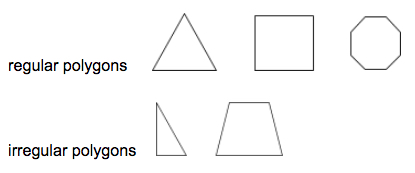A triangle is a three-sided polygon.

Examples: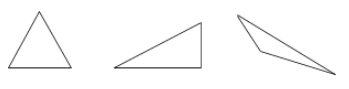A parallelogram is a type of quadrilateral where opposite sides are parallel and congruent. Opposite angles of a parallelogram are also congruent.

Examples:A rectangle is a parallelogram with four right angles.

Examples: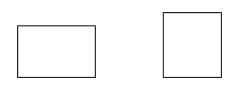A rhombus is a parallelogram in which all four sides are congruent.

Examples: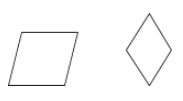A square is a parallelogram which is both a rhombus and a rectangle (all sides are congruent and all angles are right).

Examples: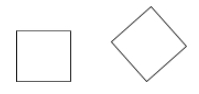A trapezoid is a quadrilateral with only one pair of parallel sides.

Examples:Polygons with more than four sides

$\begin{array}{|c|c|} \hline \textbf{Number of Sides} & \textbf{Name} \\ \hline 5 & \quad \text{pentagon}\quad \\ \hline 6 & \text{hexagon} \\ \hline 7 & \text{heptagon} \\ \hline 8 & \text{octagon} \\ \hline 9 & \text{nonagon} \\ \hline 10 & \text{decagon} \\ \hline 11 & \text{hendecagon or 11-gon}^1 \\ \hline 12 & \text{dodecagon} \\ \hline n & \text{n-gon} \\ \hline \end{array}$

$$^1$$ A polygon with 11 sides may also be called an endecagon or an undecagon.

#### Measuring Shapes

The perimeter is the total distance around a polygon. To find the perimeter, just add up the lengths of all the sides. If the polygon is regular (all sides are congruent) and the length of one side is $$s$$, then multiply the number of sides by $$s$$ to find the perimeter. For example, the perimeter of a regular pentagon is $$P_\text{reg pentagon}=5s$$

The area of a polygon is the number of square units which can fit within it. Reference these figures when reading the table.$\begin{array}{|c|c|} \hline \textbf{Shape} & \textbf{Area} \\ \hline \text{triangle} & A=\dfrac{1}{2}bh \\ \hline \text{square} & A=s^2 \\ \hline \text{rectangle} & A=l \cdot w \\ \hline \text{parallelogram} & A= b \cdot h \\ \hline \text{trapezoid} & A=\dfrac{1}{2}(b_1 + b_2) \cdot h \\ \hline \end{array}$

## All Study Guides for the Math Basics are now available as downloadable PDFs

View other purchase options